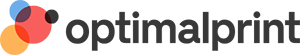Optimalprint
8.0" 10" 4806
8.0/10 (4806 reviews)

# Wedding Thank You Cards

Color
No. of Photos
Format
Orientation
Size
1004 designs Sort by:
Standard (5" x 7") - Folded
Small (5.5" x 4.25") - Folded
Small (4.25" x 5.5") - Folded
Small (4.25" x 5.5") - Folded
Small (4.25" x 5.5") - Folded
Standard (7" x 5") - Folded
Standard (7" x 5") - Folded
Small (4.25" x 5.5") - Folded
Small (5.5" x 4.25") - Folded
Small (5.5" x 4.25") - Folded
Small (4.25" x 5.5") - Folded
Small (4.25" x 5.5") - Folded
Small (4.25" x 5.5") - Folded
Standard (7" x 5") - Folded
Small (4.25" x 5.5") - Folded
Square (5.25" x 5.25") - Folded
Square (5.25" x 5.25") - Folded
Standard (5" x 7") - Folded
Standard (5" x 7") - Folded
Small (4.25" x 5.5") - Folded
Square (5.25" x 5.25") - Folded
Small (4.25" x 5.5") - Folded
Small (5.5" x 4.25") - Folded
Small (4.25" x 5.5") - Folded
Small (5.5" x 4.25") - Folded
Square (5.25" x 5.25") - Folded
Small (4.25" x 5.5") - Folded
Small (5.5" x 4.25") - Folded
Small (5.5" x 4.25") - Folded
Square (5.25" x 5.25") - Folded
Small (4.25" x 5.5") - Folded
Standard (5" x 7") - Folded
Small (5.5" x 4.25") - Folded
Small (4.25" x 5.5") - Folded
Small (4.25" x 5.5") - Folded
Small (4.25" x 5.5") - Folded
Square (5.25" x 5.25") - Folded